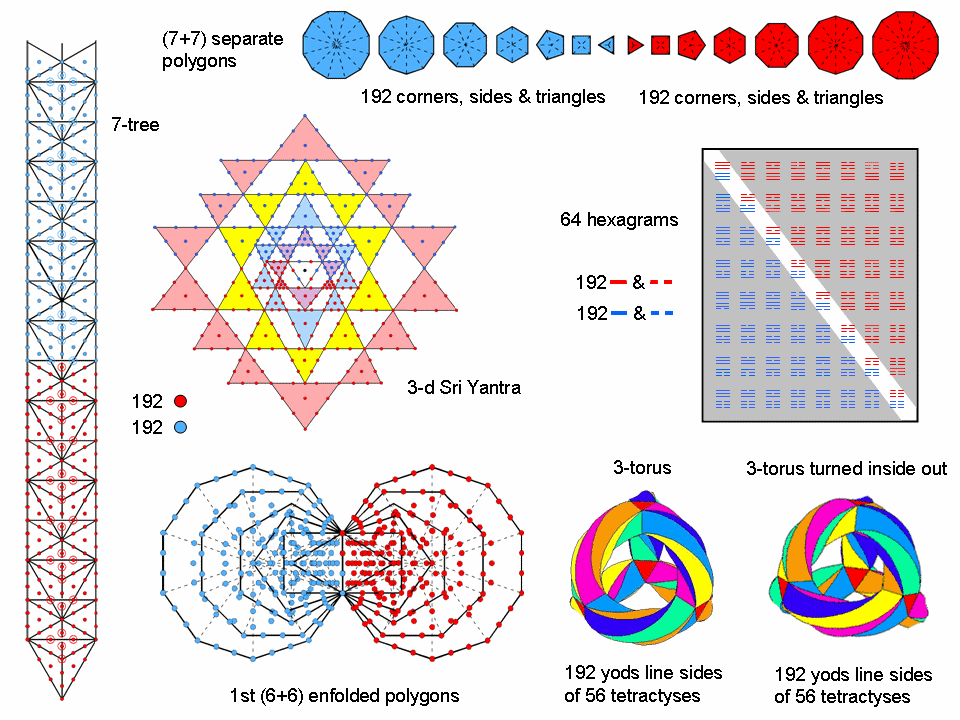<< Previous    1...   34  35    37  38  ...80    Next >>

#36 Correspondence between the 7-tree, the Sri Yantra, the (7+7) polygons, the 64 hexagrams, the first (6+6) enfolded polygons & the 3-torusThe 7-tree
This maps the physical plane (physical universe). When its 91 triangles are turned into tetractyses, it contains 380 yods. Up to the top of the 7-tree, there are two yods on either side of the central Pillar of Equilibrium that belong to the eighth Tree of Life. Hence, there are 384 yods up to this point. 192 red yods are below Chesed of the fourth Tree and 192 blue yods extend down to the Path between this SL and Geburah opposite it.

The (7+7) separate polygons
Four geometrical elements per sector surround the centre of a polygon: a corner, an outer side, an inner side & a triangle. The seven separate polygons of the inner Tree of Life have 48 sectors. Hence, (4×48=192) geometrical elements surround their centres. Both sets of seven polygons have (2×192=384) geometrical elements surrounding their 14 centres.

The first (6+6) enfolded polygons
When their 47 sectors are tetractyses, the seven enfolded polygons have 264 yods. The seventh polygon — the dodecagon — has 73 yods (69 outside their root edge). The first six enfolded polygons have (264−69=195) yods. The topmost corner of the hexagon coincides with the lowest corner of the hexagon enfolded in the next higher Tree. This means that 194 yods are intrinsic to the first six polygons enfolded in any Tree because they are unshared with the polygons enfolded in the next higher Tree. 194 is the number value of Tzadekh, the Mundane Chakra of Chesed. Two yods on the root edge shared by the polygons are associated with each set of polygons, so that 192 yods are associated with each set of the first six enfolded polygons. Both sets have 384 intrinsic yods.

The 3-d Sri Yantra
When the 43 triangles in the 3-dimensional Sri Yantra are regarded as tetractyses, there are nine yods per triangle linked together in each layer, so that the 42 tetractyses surrounding the central one have (9×42=378) yods. 189 red yods belong to the 21 triangles in one half of the Sri Yantra and 189 blue yods belong to the 21 triangles in its other half. The three corners of the central triangle symbolize the triple Godhead (Shiva, Brahma & Vishnu in Hinduism) and its centre symbolizes the Absolute. Including its six hexagonal yods (three red yods and three blue yods at the corners of two intersecting triangles), there are (189+3=192) red yods and 192 blue yods surrounding the centre of the 3-dimensional Sri Yantra, or central axis, if we include the bindu point. 384 yods are needed to construct the 43 triangles of the Sri Yantra other than the corners of its central triangle symbolizing the triple Godhead that stands outside all planes of existence.

The I Ching table of 64 hexagrams
As each of the 64 hexagrams is composed of six lines & broken lines, 384 lines & broken lines are in the table. There are 192 lines and 192 broken lines. Each diagonal half of the 8×8 array contains 192 lines & broken lines.

The {3,7} tiling of the 3-torus
The 168 automorphisms of the Klein quartic are elements of PSL(2,7) that can be represented by the {3,7} tiling of the 3-torus with 56 triangles having 168 vertices and 84 sides. Although the tetractys has no obvious bearing on the mathematics of the Klein quartic, the fact is that, when these triangles are tetractyses and the 3-torus is assembled from four triangular prisms and six square antiprisms, there are 192 yods lining their sides and 192 yods lining the sides of its version when it is turned inside-out (see the table here). This 192:192 division is characteristic of holistic systems (see The holistic pattern). The two 3-tori correspond to the lower and upper halves of the 7-tree and to the two mirror-image halves of the other sacred geometries. The inside-out 3-torus maps the 168 anti-automorphisms of the Klein quartic, so that a complete, mathematical description of its symmetries requires both tori. Moreover, the pattern of the 24 vertices of the assembled prisms and antiprisms and the 168 hexagonal yods that line their 84 sides conforms to the 24:168 divisions displayed by the other sacred geometries and by each diagonal half of the 8×8 array of hexagrams. They are analyzed in Article 43.

Such amazing consistency in both the number of degrees of freedom making up these sacred geometries and their division into various analogous subsets constitutes powerful evidence for their being equivalent representations of a unique, mathematical pattern that characterizes holistic systems. See also a discussion of the number 384 in #19.

 << Previous    1...   34  35    37  38  ...80    Next >>

Home